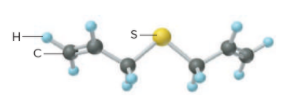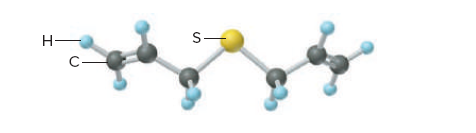# Problem: Allyl sulfide (below) gives garlic its characteristic odor. Calculate the number of carbon atoms in 35.7 g of allyl sulfide.

###### FREE Expert Solution

For this problem, we’re being asked to determine the number of carbon atoms in 35.7 g of allyl sulfideIdentify the molecular formula by counting the atoms first:

• C → 6
• S → 1
• H → 10
• Formula → C6H10S

The flow for this problem will be like this:

mass of C6H10S (Molar mass C6H10S) → mol C6H10S (mole-to-mole comparison)  mol C (Avogadro’s number) → Atoms of C

81% (80 ratings)###### Problem Details

Allyl sulfide (below) gives garlic its characteristic odor. Calculate the number of carbon atoms in 35.7 g of allyl sulfide.Frequently Asked Questions

What scientific concept do you need to know in order to solve this problem?

Our tutors have indicated that to solve this problem you will need to apply the Mole Concept concept. You can view video lessons to learn Mole Concept. Or if you need more Mole Concept practice, you can also practice Mole Concept practice problems.

What professor is this problem relevant for?

Based on our data, we think this problem is relevant for Professor Giles' class at VCU.

What textbook is this problem found in?

Our data indicates that this problem or a close variation was asked in Chemistry: The Molecular Nature of Matter and Change - Silberberg 8th Edition. You can also practice Chemistry: The Molecular Nature of Matter and Change - Silberberg 8th Edition practice problems.